Purva Huilgol — December 17, 2019

## Introduction

NeurIPS is THE premier machine learning conference in the world. No other research conference attracts a crowd of 6000+ people in one place – it is truly elite in its scope. If you want to immerse yourself in the latest machine learning research developments, you need to follow NeurIPS.

NeurIPS 2019 was the 33rd edition of the conference, held between 8th and 14th December in Vancouver, Canada. I religiously follow this conference annually and this year was no different.Every year, NeurIPS announces a category of awards for the top research papers in machine learning. These can often be difficult to understand for most folks given the advanced level of these papers.

But don’t worry! I have gone through these awesome papers and summarized the key points in this article! My aim is to help you understand the essence of each paper by breaking down the key machine learning concepts into easy-to-understand bits for our community.

Here are the three NeurIPS 2019 best paper categories I’ll cover:

• Best Paper Award
• Oustanding Directions Paper Award
• Test of Time Paper Award

Let’s dive into it!

## Best Paper Award at NeurIPS 2019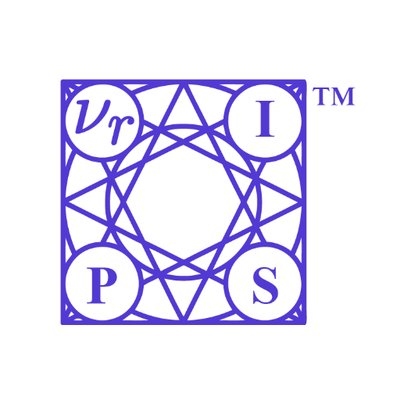And the best paper award at NeurIPS 2019 goes to:

## Distribution-Independent PAC Learning of Halfspaces with Massart Noise

This is a really great paper! It got me thinking about one of the fundamental concepts in Machine Learning: Noise and distributions. It also required a great deal of study on the paper itself and I will try to explain the gist of the paper without making it complex.

Let’s rephrase the title first. The research in this paper talks about an algorithm for learning halfspaces in the distribution-independent PAC model with Massart Noise. This algorithm is the most efficient one yet in this space.

Let us understand the key terms here.

Recall the concepts of boolean functions and binary classification. Basically,

A halfspace is a boolean function where the 2 classes (positive samples and negative samples) are separated by a hyperplane. Since the hyperplane is linear, it is also called a Linear Threshold Function (LTF).

Mathematically, a linear threshold function, or a halfspace, is a threshold function which can be represented by a linear equation of the input parameters bounded by some threshold T. A Boolean function f(x) is a linear threshold function if it has the form: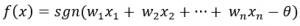where:

• w1, w2, …., wn are the weights
• x1, x2, …., xn are the features
• sgn denotes the sign function which gives the sign of a real number
• Theta is the threshold

We can also call LTFs as the perceptron (draw on your neural networks’ knowledge here!).

The PAC (Probably Approximately Correct) model is one of the standard models for binary classification.

Massart noise condition, or just Massart Noise, is when the label of each sample/record is flipped with some small probability that is unknown to the learning algorithm.

The probability of flipping is bounded by some factor n which is always lesser than 1/2. With the main goal of finding a hypothesis with a small misclassification error, various attempts have been made in the previous papers to restrict the error and the risk associated with noise in the data.

In this research on while the sample complexity was well established, a polynomial-time of (1/epsilon) was proved with an excess risk equal to the Massart noise level plus epsilon.

The paper is a great leap forward toward achieving an excess risk of only epsilon. You can read the full paper here.

## Outstanding New Directions Paper at NeurIPS 2019

NeurIPS 2019 also had a new category for a winning paper this year, called the Outstanding New Directions Paper Award. In their words:

This award is given to highlight the work that distinguished itself in setting a novel avenue for future research.

And the winner of this award is – “Uniform convergence may be unable to explain generalization in deep learning”.

One of my favorite papers this year! This paper goes on to explain, both theoretically and with empirical evidence, that the current deep learning algorithms cannot claim to explain generalization in deep neural networks. Let’s understand this in a bit more detail.

## Uniform convergence may be unable to explain generalization in Deep Learning

Large networks generalize on unseen training data pretty well despite being trained to perfectly fit randomly labeled data. But these networks should not work as well as they do when the number of features is more than the number of training samples, right?

However, they still give us state-of-the-art performance metrics. This also shows that these overparameterized models excessively depend on the parameter count and don’t account for variable batch sizes. If we go by the basic equation for generalization:

Test Error – Training Error <= Generalisation bound

For the above equation, we take the set of all hypotheses and attempt to minimize the complexity and keep these bounds as tight as possible.

The previous and current research so far has focused on tightening these bounds by concentrating on taking a relevant subset of the hypothesis class. There has also been a lot of pathbreaking research on refining these bounds, all based on the concept of Uniform Convergence.

However, this paper explains that these algorithms are either:

• Too large and their complexity grows with the parameter count, or
• Small, but have been developed on a modified network

The paper defines a set of criteria for generalization bounds and demonstrates a set of experiments to prove how uniform convergence cannot fully explain generalization in deep learning.

The generalization bounds:

1. Must be ideally <1 (vacuous)
2. Become smaller with increasing width
3. Apply to the network learned by SGD (Stochastic Gradient Descent)
4. Increase with the proportion of randomly flipped training labels
5. Should vary inversely with dataset size

The experiment I mentioned earlier has been done on the MNIST dataset with three types of overparameterized models (all trained on the SGD algorithm):

1. A linear classifier
2. A wide neural network with ReLU
3. A neural network of infinite width with frozen hidden weights

The paper goes on to demonstrate different hyperparameter settings for varying training set sizes.

One really interesting observation I saw is that though the test set error decreases with an increase in training set size, the generalization bounds, in fact, show an increase.

What if the network is just memorizing the data points we keep adding to the training set?

Let’s take an example given by the researchers. For a classification task on a dataset with 1000 dimensions, an overparameterized model with 1 hidden layer ReLU and 100k units is trained using SGD. Increasing the training set size improves the generalization and reduces the test set error.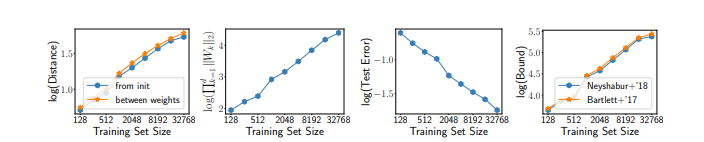However, despite the generalization, they prove that the decision boundary is quite complex. This is where they go against the idea of uniform convergence.

Thus, uniform convergence cannot completely explain the generalization, even for linear classifiers. In fact, it can actually be considered a contributing factor towards an increase in the bounds when increasing the sample size!

While previous research had driven the direction of developing deep networks towards being algorithm-dependent (in order to stick to uniform convergence), this paper proposes a need for developing algorithm-independent techniques that don’t restrict themselves to uniform convergence to explain generalization.

We can clearly see why this machine learning research paper has received an award for Outstanding New Directions Paper at NeurIPS 2019.

The researchers have shown that merely uniform convergence is not enough to explain generalization in deep learning. Also, it is not possible to achieve small bounds satisfying all the 5 criteria. This has opened a whole new research area into exploring other tools which might explain generalization.

You can access and read the full paper here.

## Test of Time Award at NeurIPS 2019

Each year, NeurIPS also gives an award to a paper presented at the conference 10 years ago and that has had a lasting impact on the field in its contributions (and is also a widely popular paper).

This year, the aptly named Test of Time award has been given to the “Dual Averaging Method for Regularized Stochastic Learning and Online Optimization” by Lin Xiao. This research is based on the fundamental concepts which built the very foundations of modern machine learning as we know it.

## Dual Averaging Method for Regularized Stochastic Learning and Online Optimization

Let’s break down the four key concepts covered in this awesome paper:

1. Stochastic Gradient Descent: Stochastic Gradient Descent has been formally established as the method for optimization in machine learning. This can be achieved by the following stochastic optimization. Recall SGD and the equation for stochastic optimization for very large samples – here, w is the weight vector and z is the input feature vector. For t = 0, 1, 2….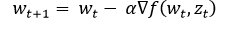2. Online Convex Optimisation: Another pathbreaking research. This was simulated as a game where the player will try to predict a weight vector and the resulting loss would be calculated at each t. The main aim is to minimize this loss – the result is very similar to how we optimize using stochastic gradient descent
3. Compressed Learning: This includes Lasso Regression, L1 Regularized Least-Squares, and other mixed regularization schemes
4. Proximal Gradient Method: Compared to the earlier techniques, this was a much faster method for reducing the loss and still retaining the convexity

While the previous research developed an efficient algorithm converging to O(1/t), the sparsity of data was one factor that had been neglected until then. This paper proposed a new regularizing technique, called the Regularised Dual Averaging Method (RDA) for solving online convex optimization problems.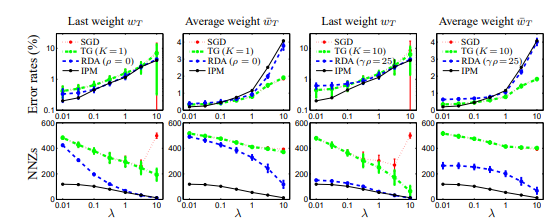At that time, these convex optimization problems were not efficient, particularly in terms of scalability.

This research proposed a novel method of Batch Optimization. This means that only some independent samples are made available initially, and the weight vector is calculated based on those samples (at current time t). The loss with respect to the current weight vector is calculated along with a subgradient. This is used again in the iteration (at time t+1).

At that time, this method had achieved much better results for the sparse MNIST dataset than the SGD and other prevalent techniques. In fact, with an increase in sparsity, the RDA method had demonstrably better results as well.

The reason this was awarded the test of time paper is evident in the different papers which studied the above method further, like manifold identification, accelerated RDA, etc.

You can find the complete paper here.

## End Notes

NeurIPS 2019 was an extremely educational and inspiring conference again. I was especially intrigued by the New Directions Paper award and how it tackled the problem of generalization in deep learning.

Which machine learning research paper caught your eye? Did you like any other paper than you would want to try out or that really inspired you? Let me know in the comments section below.

All of the talks, including the spotlights and showcases, were broadcast live by the NeurIPS team. You can find links to the recorded sessions here.###### Purva Huilgol

Trainee Data Scientist at Analytics Vidhya. Pursuing Masters in Data Science from the University of Mumbai, Dept. of Computer Science. ML and NLP enthusiast.